Ask your WordPress questions! Pay money and get answers fast!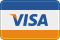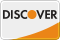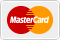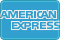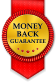# Create a Genesis ShortCode from this? WordPress

• REFUNDED

I am using the following code on a site:
```<div class='x-rating-box shortcode-left'> <?php // get the star ratings in an array of arrays \$ratings = myrp_api_editor_ratings(); if(count(\$ratings)>0) { ?> <h3>Editor's Ratings</h3> <div class='x-rating-box-inner'> <?php foreach(\$ratings as \$rating) { // output the category name, and then the star images, disabled for the rating. echo "<div class='x-rating-stars' style='float:right;'>" . myrp_get_rating_fixed_row(myrp_get_rating_category(myrp_get_category_id(\$rating)), \$rating) . "</div>" . "<div class='x-rating-title'>" . \$rating . "</div>" ; } ?> </div> <?php } ?> <?php // get the star ratings in an array of arrays \$ratings = myrp_api_ratings(); if(count(\$ratings)>0) { ?> <h3>Your Ratings</h3> <div class='x-rating-box-inner'> <?php foreach(\$ratings as \$rating) { // output the category name, and then the star images, disabled for the rating. echo "<div class='x-rating-stars' style='float:right;'>" . myrp_get_rating_fixed_row(myrp_get_rating_category(myrp_get_category_id(\$rating)), \$rating) . "</div>" . "<div class='x-rating-title'>" . \$rating . "</div>" ; } // output the number of positive and negative ratings. //\$positive_negative = myrp_api_positive_negative(); // echo "<div class='posneg'>+" . \$positive_negative['positive'] . " positive &mdash; " . \$positive_negative['negative'] . " negative</div>"; ?> </div> <?php } ?> </div>```

It prints three things:
1- Editors Rating
2- Users Rating
3- The # of positive and negative reviews received

If there was a way to make a shortcode that replicated the same functionality, it would make my life much easier.

2013-08-02

Try this

use this shortcode

[wpqratings]

```function wpq_rating_sc( ) { \$ret = '<div class="x-rating-box shortcode-left">'; // get the star ratings in an array of arrays \$ratings = myrp_api_editor_ratings(); if(count(\$ratings)>0) { \$ret .= "<h3>Editor's Ratings</h3> <div class='x-rating-box-inner'>"; foreach(\$ratings as \$rating) { // output the category name, and then the star images, disabled for the rating. \$ret = "<div class='x-rating-stars' style='float:right;'>" . myrp_get_rating_fixed_row(myrp_get_rating_category(myrp_get_category_id(\$rating)), \$rating) . "</div>" . "<div class='x-rating-title'>" . \$rating . "</div>"; } \$ret .= "</div>"; } // get the star ratings in an array of arrays \$ratings = myrp_api_ratings(); if(count(\$ratings)>0) { \$ret .= "<h3>Your Ratings</h3> <div class='x-rating-box-inner'>"; foreach(\$ratings as \$rating) { // output the category name, and then the star images, disabled for the rating. \$ret .= "<div class='x-rating-stars' style='float:right;'>" . myrp_get_rating_fixed_row(myrp_get_rating_category(myrp_get_category_id(\$rating)), \$rating) . "</div>" . "<div class='x-rating-title'>" . \$rating . "</div>" ; } // output the number of positive and negative ratings. //\$positive_negative = myrp_api_positive_negative(); // echo "<div class='posneg'>+" . \$positive_negative['positive'] . " positive &mdash; " . \$positive_negative['negative'] . " negative</div>"; \$ret .= "</div>"; } \$ret .= "</div>"; return \$ret; } add_shortcode( 'wpqratings', 'wpq_rating_sc' );```